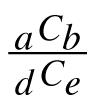Home > CCA2 > Chapter 12 > Lesson 12.1.2 > Problem12-36

12-36.

If five cards are dealt from a shuffled deck of playing cards: Homework Help ✎

1. What is the probability of getting a five-card hand that is all red?

There are $26$ red cards, choose $5$. The total possible hands come from $52$ cards, choose $5$.

$0.0253$

2. Express your solution to part (a) in the form: $\frac { a C _ { b } } { d C _ { e } }$.

See the hint in part (a).

3. What is the probability of getting a five-card hand that is all clubs?

$0.000495$

4. Express your solution to part (c) in the form.

There are $13$ clubs, choose $5$.

5. What is the probability of getting 5 cards from any one suit?

You will need to add the probabilities of all hearts, all diamonds, all clubs, and all spades.